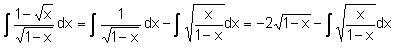Click to Chat

1800-1023-196

+91-120-4616500

CART 0

• 0

MY CART (5)

Use Coupon: CART20 and get 20% off on all online Study Material

ITEM
DETAILS
MRP
DISCOUNT
FINAL PRICE
Total Price: Rs.

There are no items in this cart.
Continue Shopping```Standard Substitutions

•For terms of the form x2 + a2 or √x2 + a2, put x = a tanθ or a cotθ

For terms of the form x2 - a2 or √x2 – a2 , put x = a sec θ or a cosecθ

(A)For terms of the form a2 - x2 or √x2 + a2, put x = a sin θ or a cosθ

• If both √a+x, √a–x,  are present, then put x = a cos θ.

• For the type √(x–a)(b–x), put x = a cos2θ + b sin2θ

• For the type (√x2+a2±x)n or (x±√x2–a2)n, put the expression within the bracket = t.

• For the type(n ∈ N, n> 1), put x+b/x+a = t

• For 1/(x+a)n1 (x+b)n2, n1,n2 ∈ N (and > 1), again put (x + a) = t (x + b)

Example -6: EvaluateSolution:= I2

Put 1 –x = t2

dx = 2t dt

I = –√1–x + √x√1–x + sec–1 √1–x + k

Example -7: Evaluate .∫ dx / (x+1)6/5 (x–3)4/5To read more, Buy study materials of Indefinite integral comprising study notes, revision notes, video lectures, previous year solved questions etc. Also browse for more study materials on Mathematics here.
```### Course Features

• 731 Video Lectures
• Revision Notes
• Previous Year Papers
• Mind Map
• Study Planner
• NCERT Solutions
• Discussion Forum
• Test paper with Video Solution